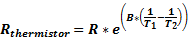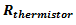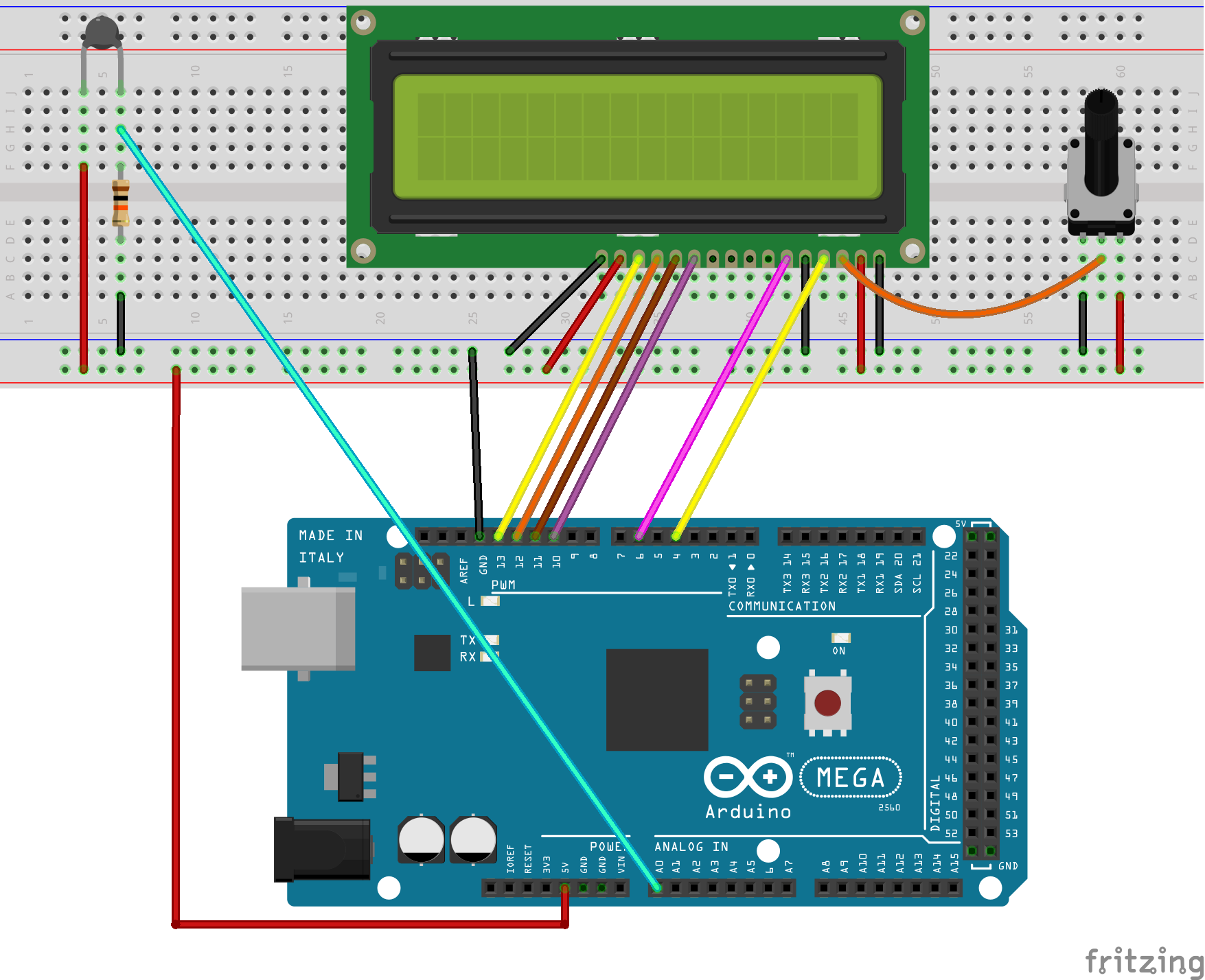Index>Arduino>Ultimate Starter Kit for Arduino Mega2560-V2.0>Lesson 16 Use a thermistor to measure the temperature
No related product information!

# Lesson 16 Use a thermistor to measure the temperature

### 1515

Overview

In this lesson, we will learn how to use a thermistor to collect temperature by programming Arduino. The information which a thermistor collects temperature is displayed on the LCD1602.

Requirement

- 1* Arduino MEGA 2560

- 1* USB Cable

- 1* LCD1602

- 1* 10KΩ Potentiometer

- 1* 10KΩ Resistor

- 1* Thermistor

- Several Jumper Wires

Principle

A thermistor is a type of resistor whose resistance varies significantly with temperature, more so than in standard resistors. We are using MF52 NTC thermistor type. BTC thermistor is usually used as a temperature sensor.

MF52 thermistor key parameters:

B-parameter：3470.

25 resistor：10KΩ.

The relationship between the resistance of thermistor and temperature is as follows:: The resistance of thermistor at temperature T1

R: The nominal resistance of thermistor at room temperature T2;

e: 2.718281828459

B: It is one of the important parameters of thermistor;

T1: The Kelvin temperature that you want to measure.

T2: At the condition of room temperature 25 ℃ (298.15K), the standard resistance of MF52 thermistor is 10K;

Kelvin temperature = 273.15 (absolute temperature) + degrees Celsius;

After transforming the above equation, we can get to the following formula:Procedures

1. Build the circuit2. Program_16_thermistor.ino

3. Compile the program and upload to Arduino MEGA 2560 board

Now, you can see the temperature which is collected by thermistor on the LCD1602.Summary

By learning this lesson, I believe you have learned to use a thermistor to measure temperature. Next, you can use a thermistor to produce some interesting applications.